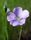## Time difference of 2 numeric variable in PROC SQL

Hello guys,

I want to calcuate and express the time difference between two numeric variables in PROC SQL.

For example, start_time is 103027. It means that the start time is 10:30:27

and end_time is 114037. It means that the end time is 11:40:37.

Both variables are numeric type and their calculated time differnece is  1 hour 10 minutes 10 seconds.

How can I express PROC SQL in this logic?

If you have any questions, please leave comment below.

4 REPLIES 4LinusH
Tourmaline | Level 20

## Re: Time difference of 2 numeric variable in PROC SQL

I think it's best to convert the fields to SAS time format.

For that, you should use SAS time informats.

Once done, a simple subtraction will give you the difference in seconds, which in turn can apply a format so you can view it with minutes and hours.

https://documentation.sas.com/doc/en/pgmsascdc/9.4_3.5/leforinforref/n0verk17pchh4vn1akrrv0b5w3r0.ht...

Data never sleeps

## Re: Time difference of 2 numeric variable in PROC SQL

You should provide some example values and the time interpretation when you have

1) hour is less than 10 and minutes and seconds greater than 10

2) hour is greater than or equal to 10 and minutes less than 10 and seconds greater than 10

3) hour is greater than or equal to 10 and minutes greater than 10 and seconds less than 10

The above goes to what may be needed to create a valid SAS time value. If you have 10203 and in one place might be 1:02:03 (hours less than 10), 10:02:30 (minutes less than 10) or 10:20:03 (seconds less than 10) in another this process may not be doable at all. With the oddities I have seen people call times over the years I really want good examples before writing any code.

Note: it may be easier to read from text with a proper informat than to convert existing numerical values that may have had information removed.

Are these values from a 12 hour clock or 24 hour clock? If 12 hour then you need to tell us how to know if 10:30:27 is AM or PM. Because without that information then your result could be 12 hours off. Or even if they are the same day?

## Re: Time difference of 2 numeric variable in PROC SQL

As @LinusH wrote, it is easy if you convert the values to SAS time values. In SQL, it can be done like this:

``````proc sql;
create table want as select
hms(int(start_time/10000),mod(int(start_time/100),100),mod(start_time,100)) as start_time format=time8.,
hms(int(end_time/10000),mod(int(end_time/100),100),mod(end_time,100)) as end_time format=time8.,
calculated end_time - calculated start_time as elapsed_time format=time8.
from have;
quit;
``````

## Re: Time difference of 2 numeric variable in PROC SQL

I could not think of a simpler solution than one very similar to what @s_lassen has provided.

So to the original question from @Jack1208 , you would be wise (if at all possible) to not store hours/minutes/seconds as an integer such as 103027 indicating 10 hours, 30 minutes, 27 seconds. Most databases and most programming languages have built in ways to store hours/minutes/seconds, better to store your data in one of those supported ways, rather than the way it is stored as 103027.

--
Paige Miller
Discussion stats
• 4 replies
• 261 views
• 0 likes
• 5 in conversation